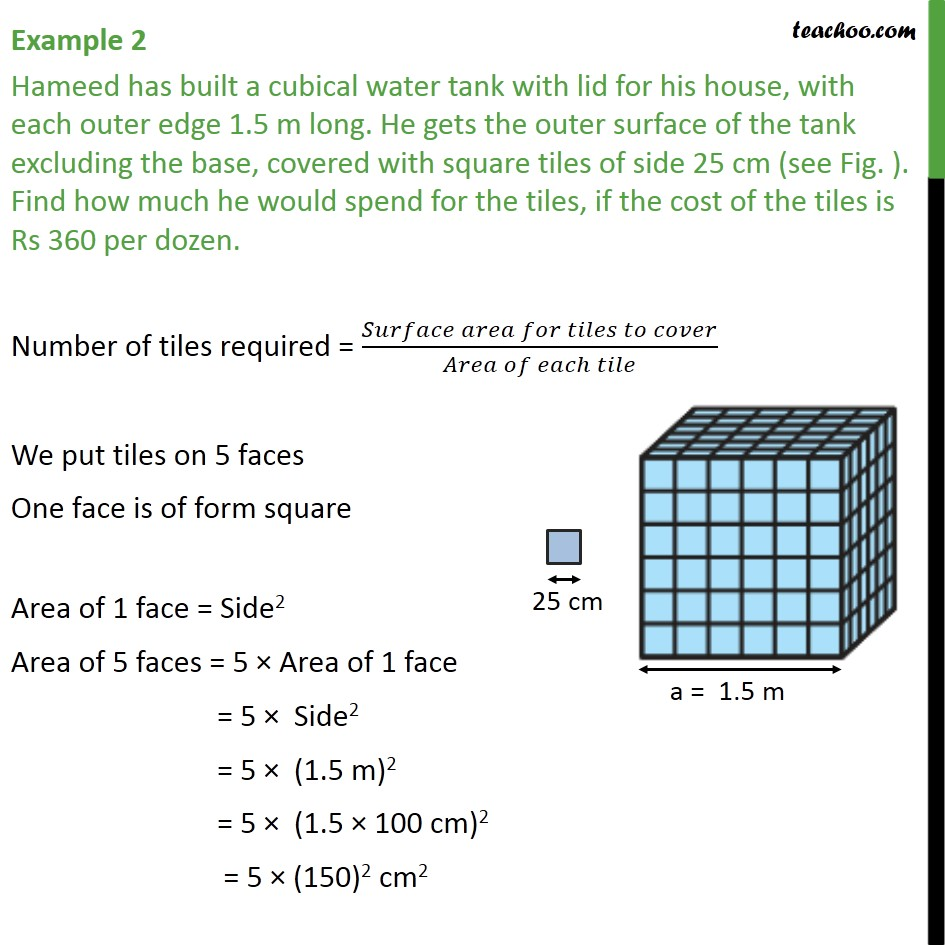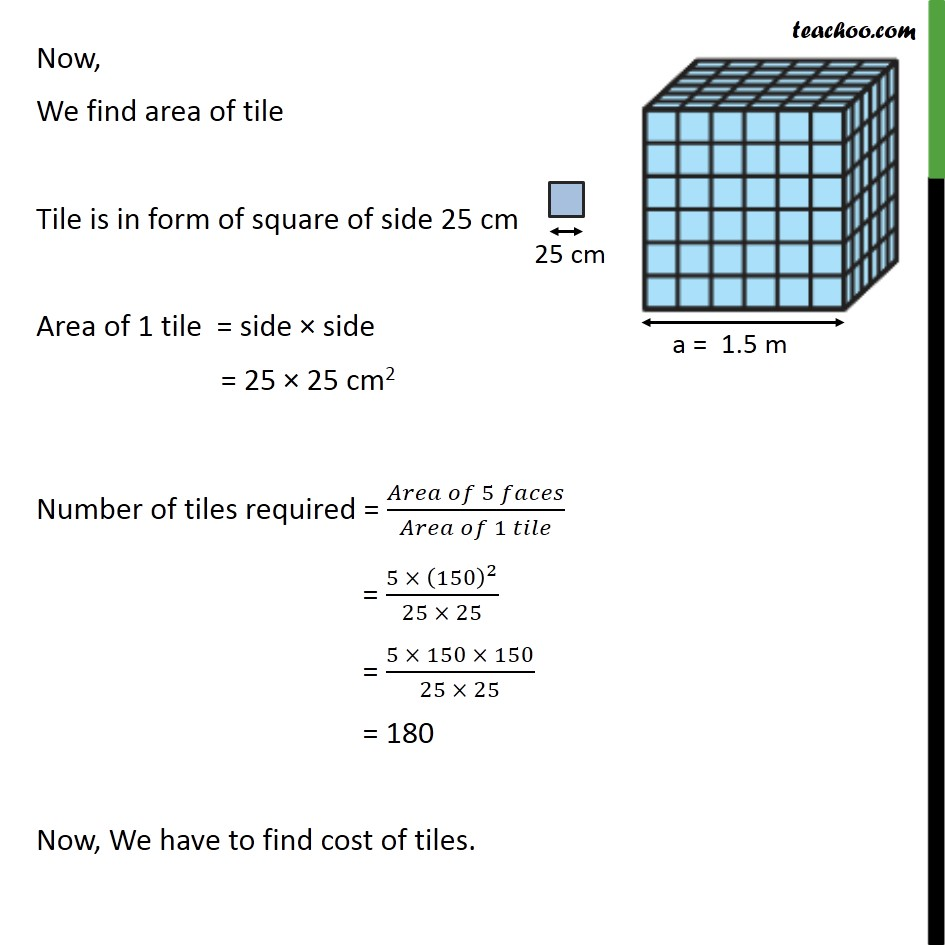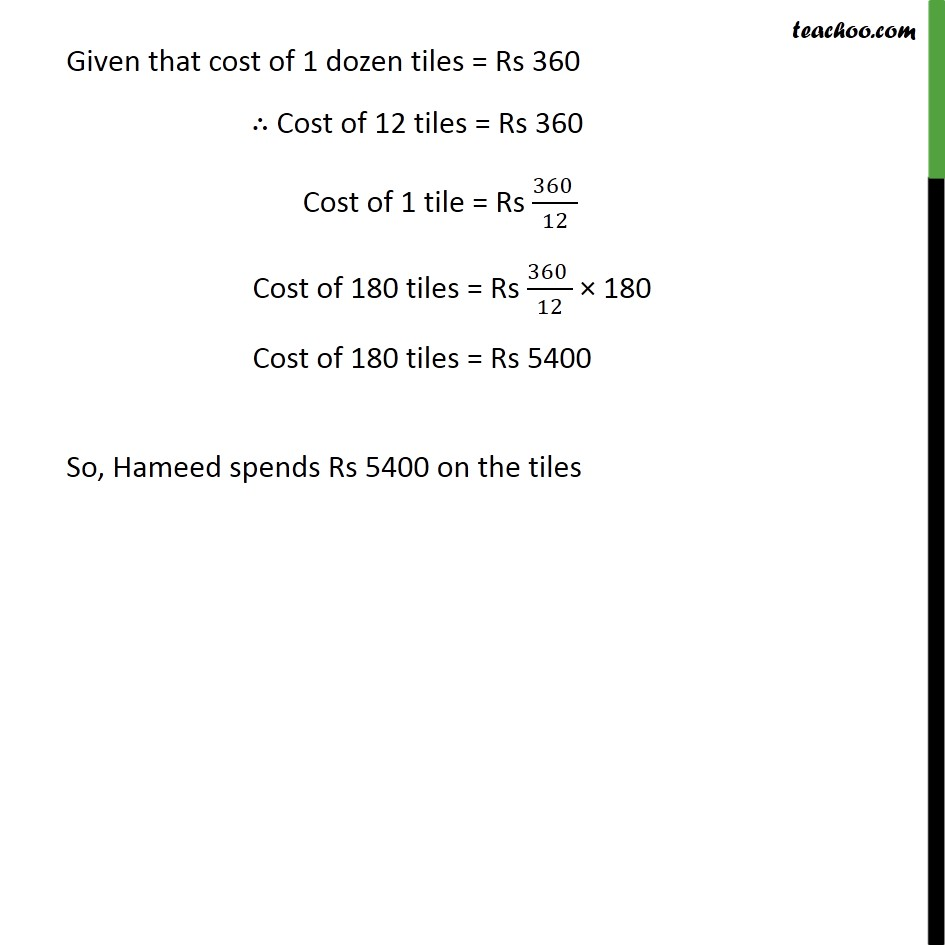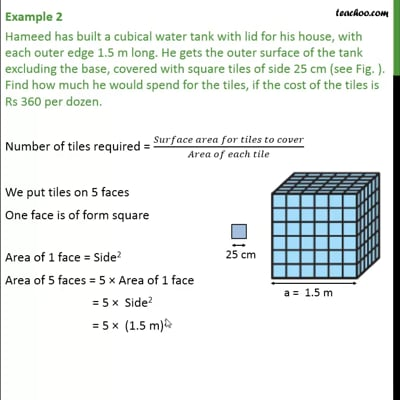Area Of Cube/ Cuboid

Chapter 13 Class 9 Surface Areas and Volumes
Concept wiseThis video is only available for Teachoo black users

Introducing your new favourite teacher - Teachoo Black, at only ₹83 per month

### Transcript

Example 2 Hameed has built a cubical water tank with lid for his house, with each outer edge 1.5 m long. He gets the outer surface of the tank excluding the base, covered with square tiles of side 25 cm (see Fig. ). Find how much he would spend for the tiles, if the cost of the tiles is Rs 360 per dozen. Number of tiles required = ( )/( ) We put tiles on 5 faces One face is of form square Area of 1 face = Side2 Area of 5 faces = 5 Area of 1 face = 5 Side2 = 5 (1.5 m)2 = 5 (1.5 100 cm)2 = 5 (150)2 cm2 Now, We find area of tile Tile is in form of square of side 25 cm Area of 1 tile = side side = 25 25 cm2 Number of tiles required = ( 5 )/( 1 ) = (5 (150)^2)/(25 25) = (5 150 150)/(25 25) = 180 Now, We have to find cost of tiles. Given that cost of 1 dozen tiles = Rs 360 Cost of 12 tiles = Rs 360 Cost of 1 tile = Rs (360 )/12 Cost of 180 tiles = Rs (360 )/12 180 Cost of 180 tiles = Rs 5400 So, Hameed spends Rs 5400 on the tiles